ncert textbook

# Knowing Our Numbers Class 6 Extra Questions Maths Chapter 1

Extra Questions for Class 6 Maths Chapter 1 Knowing Our Numbers

### Knowing Our Numbers Class 6 Extra Questions Very Short Answer Type

Question 1.
Write the smallest three digit number whose value does not change on reversing its digits.
Solution:
The required number is 101.

Question 2.
Write the greatest three digit number which does not change on reversing its digits.
Solution:
The required number is 999.

Question 3.
What must be added to 203 to get a number whose digits are reversed of the given number?
Solution:
The number obtained by reversing the digits of 203 = 302.
∴ Difference = 302 – 203 = 99
Hence, the required number is 99.

Question 4.
Write the following in Roman numerals:
(a) 72
(b) 38
Solution:
(a) 72 = LXXII
(b) 38 – XXXVIII

Question 5.
Write 438 in its expanded form.
Solution:
438 = 4 x 100 + 3 x 10 + 8.

Question 6.
Write the greatest five-digit number using the digits 4, 2 and 0.
Solution:
The greatest five-digit number using the digits 4, 2 and 0 is 44420.

Question 7.
The capacity of a water tank is 300 litres. Express its capacity in millilitres.
Solution:
We know that
1 litre = 1000 mL
∴ 300 litres = 300 x 1000 mL = 3,00,000 mL
Hence, the capacity of water tank = 3 lakh millilitres.

Question 8.
What is the successor of greatest 6-digit number?
Solution:
Greatest 6-digit number = 999999
Successor of it = 999999 + 1 = 1000000
i. e., smallest 7-digit number.
Hence, the required successor = 10,00,000.

Question 9.
What is the place value of 7 in 1743?
Solution:
Let us write 1743 in its expanded form
1743 = 1000 + 700 + 40 +3
Place value of 7 = 700
Hence, the place value of 7 = 700.

### Knowing Our Numbers Class 6 Extra Questions Short Answer Type

Question 10.
Of 7,12,540 and 71,25,400 which number is greater and by how much?
Solution:
Since 71,25,400 is a seven-digit number and 7,12,540 is a six-digit number.
So 71,25,400 is greater than 7,12,540.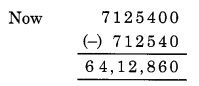Hence 71,25,400 is greater than 7,12,540 by 64,12,860.

Question 11.
Write the smallest and the greatest 5-digit numbers using the digits 0,2,4,6,8 (Repetition of digits is not allowed).
Solution:
Given digits are 0, 2, 4, 6, 8
5 – digit greatest number = 86420;
5 – digit smallest number = 20468.

Question 12.
Write the following numbers in ascending order. How many of them are even numbers?
63,854, 63,584, 65,348, 68,543, 64,835
Solution:
The given numbers are 63,854, 63,584, 65,348, 68,543 and 64,835.
Ascending order is 63,584 ; 63,854 ; 64,835 ; 65,348 ; 68,543
Even numbers are 63,584, 63,854 and 65,348.

Question 13.
Round the given numbers to the nearest tens.
(a) 48
(b) 59
(c) 64
(d) 215
Solution:
Given number Rounded off to tens
(a) 48 → 50
(6) 59 → 60
(c) 64 → 60
(d) 215 → 220

Question 14.
Estimate the following products:
(а) 86 x 316
(b) 898 x 786
Solution:
(a) 86 x 316
∵ 86 → 90 [Rounding off to tens] and 316 → 320 [Rounding off to tens]
So, the estimated product is 90 x 320 = 28800

(b) 898 x 786
∵ 898 → 900 [Rounding off to hundreds] and 786 → 800 [Rounding off to hundreds]
So, the estimated product is 900 x 800 = 720000.

Question 15.
Divide 2,63,175 by 275.
Solution:
We have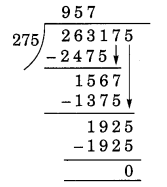Hence, quotient = 957 and remainder = 0.

Question 16.
A student multiplied 3759 by 231 instead of multiplying by 213. How much was his product greater than the correct product?
Solution:
First Method:
(3759 x 231)-(3759 x 213) = 868329 – 800667 = 67662
Second Method: 3759 x (231 – 213) = 3759 x 18 = 67662
Hence, the product difference is 67662.

Question 17.
Estimate: 25,148 + 7394 + 9343 + 752
Solution:
Estimated values are
25,148     →    25100
7394        →     7400
9343       →      9300
752          →      800
So, the estimated sum is 25100 + 7400 + 9300 + 800 = 42600
Hence, the estimated sum is 42600.

Question 18.
Write all the even numbers between 90 and 100 in Roman Numerals.
Solution:
Even numbers between 90 and 100, we have 92, 94, 96, 98.
∴ 92 = XCII,
94 = XCIV,
96 = XCVI,
98 = XCVIII

### Knowing Our Numbers Class 6 Extra Questions Long Answer Type

Question 19.
Write the missing digits in the following sums:Solution: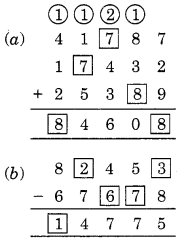Question 20.
Write Hindu-Arabic numerals for:
(a) LXXXVI
(b) LXXV
(c) XCIX
(d) XCI
Solution:
(a) LXXXVI = 50 + 30 + 6 = 86
(b) LXXV = 50 + 20 + 5 = 75
(c) XCIX = (100 – 10) + 9 = 99
(d) XCI = (100 – 10) + 1 = 91

Question 21.
The distance between the school and Reena’s house is 1 km 480 m. Everyday she walks both ways. What distance does she cover in 6 days of a week?
Solution:
Distance covered when she walks one way = 1 km 480 m = 1480 m
Therefore, the distance covered when she walk both ways in a day = 1480 x 2 m = 2960 m
Total distance covered by Reena in 6 days = 2960 x 6 m = 17760 m or 17 km 760 m.

Question 22.
Simplify: 36 ÷ [5 + {4 x 5 ÷ 2}]
Solution:
Given:
36 ÷ [5 + {4 x 5 ÷ 2}]
Using B, O, D, M, A, S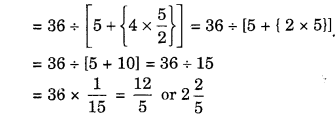Question 23.
To stitch a pant 1 m 15 cm cloth is needed. Out of 36 m cloth, how many pants can be stitched and how much cloth will remain?
Solution:Cloth required to stitch 1 pant = 1 m 15 cm
= 100 cm + 15 cm [∵ 1 m = 100 cm]
= 115 cm
Total cloth = 36 m = 36 x 100 cm = 3600 cm
Therefore number of pants stitched =$\frac { 3600 }{ 115 }$
Hence, 31 pants can be stitched and cloth left over is 35 cm.

Question 24.
Write each of the following numbers in figures:
(a) Eighty-one million four hundred twelve thousand six hundred fifty.
(b) Twenty million three hundred eighty thousand one hundred.
(c) Ninety million nine.
(d) Forty-nine million seven hundred eighty two thousand fifty eight.
(e) Six millions three hundred fifty-two thousand nine hundred forty-six.
(f) Seven crore twenty-three lakh eighty-six thousand, five hundred ninety-four.
(g) Fifty crore forty lakh sixty thousand nine.
(h) Nineteen crore, ninety lakh, fourteen thousand, six hundred eighty.
Solution:
In words                                                                                                                         In figure
(а) Eighty-one millions four hundred twelve thousand, six hundred fifty.     81,412,650
(b) Twenty million three hundred eighty thousand one hundred                     20,380,100
(c) Ninety million nine                                                                                               90,000,009
(d) Forty-nine million seven hundred eighty-two thousand fifty-eight           49,782,058
(e) Six-millions three hundred fifty-two thousand nine hundred forty-six    6,352,946
(f) Seven crore, twenty-three lakh eighty-six thousand five hundred ninety-four 7,23,86,594
(g) Fifty crore forty lakh sixty thousand nine                                                        50,40,60,009
(h) Nineteen crore ninety lakh fourteen thousand six hundred eighty.           19,90,14,680

Question 25.
Write True/False for the following statements:
(a) Roman symbol X cannot be repeated more than three times
(b) VXXX = 25 …….. .
(c) Estimate value of274 rounding off to nearest hundreds = 200 …….
(d) I and X can repeat at the most three times …….
(e) V, L and D are neither, repeated nor written to the left of greater value symbol ……..
(f) There are six basic symbols in Roman Numeration system ……..
Solution:
(a) True
(b) False
(c) False
(d) True
(e) True
(f) False.

### Knowing Our Numbers Class 6 Extra Questions Higher Order Thinking Skills (HOTS)

Question 26.
There are two factories located at place P and the other at place Q. From these factories, a certain commodity is to be delivered to each of the depots situated at A, B and C. Weekly production of commodity by P and Q are 120 kg and 150 kg respectively. Weekly requirement of commodity by A, B and C are 80 kg, 90 kg and 100 kg respectively. P delivers 60 kg to A, 40 kg to B and 20 kg to C. How much amount of the commodity should Q deliver to A, B and C to meet their requirement? If the rate of the commodity is ? 20 per kg, find the total amount to be paid to P and Q.
Solution: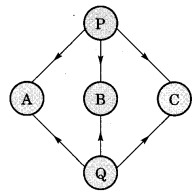Amount of commodity delivered by P to A = 60 kg
Amount of commodity delivered by Q to A = 80 – 60 = 20 kg
Amount of commodity delivered by P to B = 40 kg
Amount of commodity delivered by Q to B – 90 – 40 = 50 kg
Amount of commodity delivered by P to C = 20
Amount of commodity delivered by Q to C = 100 – 20 = 80 kg.
Now Amount of money to be paid to P by A, B and C = ₹( 60 x 20 + 40 x 20 + 20 x 20)
= ₹ (1200 + 800 + 400)
= ₹ 2400
and amount of money to be paid to Q by A, B and C
= ₹ (20 x 20 + 50 x 20 + 80 x 20)
= ₹ (400 + 1000 + 1600) = ₹ 3000
Hence, the total amount
= ₹ 2400 + ₹ 3000 = ₹ 5400.